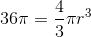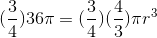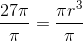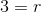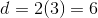# High School Math : How to find the diameter of a sphere

## Example Questions

### Example Question #1 : How To Find The Diameter Of A Sphere

What is the diameter of a sphere with a volume of?Explanation:

To find the diameter of a sphere we must use the equation for the volume of a sphere to find the radius which is half of the diameter.

The equation is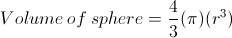First we enter the volume into the equation yielding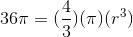We then divide each side by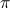to get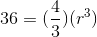We then multiply each side by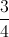to get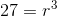We then take the cubic root of each side to solve for the radius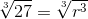The radius is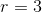We then multiply the radius by 2 to find the diameter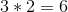The answer for the diameter is.

### Example Question #2 : How To Find The Diameter Of A Sphere

The volume of a sphere is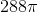. What is the diameter?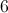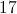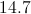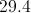Explanation:

To find the diameter of the sphere, we need to find the radius.

The volume of a sphere is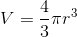.

Plug in our given values.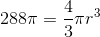Notice that the's cancel out.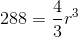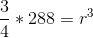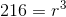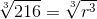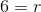The diameter is twice the radius, so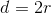.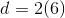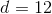### Example Question #3 : How To Find The Diameter Of A Sphere

If the surface area of a sphere is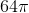, find the diameter of this sphere.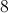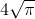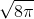Explanation:

The standard equation to find the surface area of a sphere is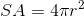wheredenotes the radius. Rearrange this equation in terms of: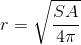Substitute the given surface area into this equation and solve for the radius and then double the radius to get the diameter: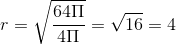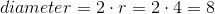### Example Question #4 : How To Find The Diameter Of A Sphere

Given that the volume of a sphere is, find the diameter.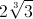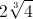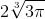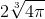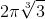Explanation:

The standard equation to find the volume of a sphere iswheredenotes the radius. Rearrange this equation in terms of: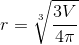Substitute the given volume into this equation and solve for the radius. Double the radius to find the diameter: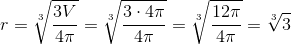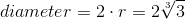### Example Question #5 : How To Find The Diameter Of A Sphere

What is the diameter of a sphere with a volume of?Explanation:

The volume of a sphere is determined by the following equation: